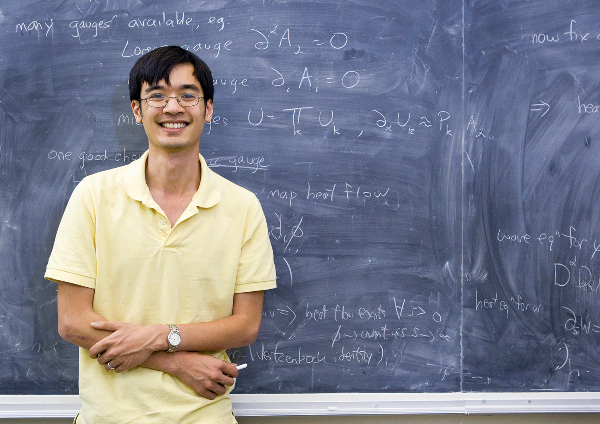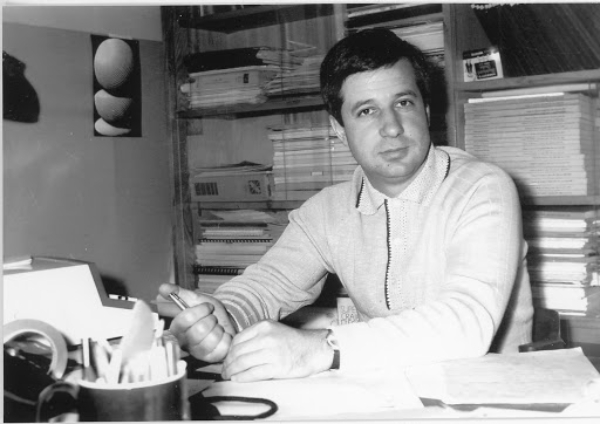# Sendov’s conjecture for sufficiently high degree polynomials

## Terence Tao, University of California, Los Angeles (UCLA) Winner of the Fields Medal 2006, the Breakthrough Prize in Mathematics, the Crafoord Prize 2012Acad. B. Sendov was the founding director of ICMS-Sofia. We celebrate his memory with a talk we hope will make him happy.

Abstract: In 1958, Blagovest Sendov made the following conjecture: if a polynomial f of degree n 2 has all of its zeroes in the unit disk, and a is one of these zeroes, then at least one of the critical points of f lies within a unit distance of a. Despite a large amount of effort by many mathematicians and several partial results (such as the verification of the conjecture for degrees n 8), the full conjecture remains unresolved. In this talk, we present a new result that establishes the conjecture whenever the degree n is larger than some sufficiently large absolute constant n0. A result of this form was previously established in 2014 by Degot assuming that the distinguished zero a stayed away from the origin and the unit circle. To handle these latter cases we study the asymptotic limit as n → ∞ using techniques from potential theory (and in particular the theory of balayage), which has connections to probability theory (and Brownian motion in particular). Applying unique continuation theorems in the asymptotic limit, one can control the asymptotic behavior of both the zeroes and the critical points, which allows us to resolve the case when a is near the origin via the argument principle, and when a is near the unit circle by careful use of Taylor expansions to gain fine asymptotic control on the polynomial f.